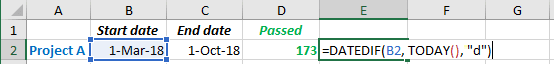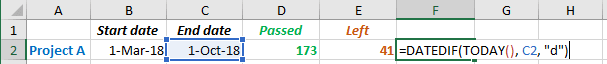# How to calculate how many days left to or passed from the specific date in Excel

Excel
For some projects or tasks, you might need to calculate how many days, months, or years left to some date or how many days, months, or years passed from some date till today. Excel proposes a very useful formula that can help you to calculate that very fast.

The formula DATEDIF calculates days, months or years between two dates:

= DATEDIF (<start date>, <end date>, unit)

The parameter unit can be:

• "D" - The number of days between to dates.

For example:

• To calculate how many days passed from the date:

= DATEDIF (<start date>, TODAY (), “d”)Notes:

1. You can use a simpler formula that yields the same result:

= TODAY () - <start date>

2. The formula TODAY () calculates the current date. Sure, you can enter the specific date to calculate the differences, see Calculating the Number of Days between two dates.
• To calculate how many days left to the date:

= DATEDIF (TODAY (), <end date>, “d”)Note: You can use a simpler formula that yields the same result:

= <end date> - TODAY ().

See also this tip in French: Comment calculer le nombre de jours restants ou passés à partir de la date spécifique dans Excel.

If you have any questions or suggestions, please feel free to ask OfficeToolTips team.

We use cookies to personalise content and ads, to provide social media features and to analyse our traffic. We also share information about your use of our site with our social media, advertising and analytics partners who may combine it with other information you’ve provided to them or they’ve collected from your use of their services.